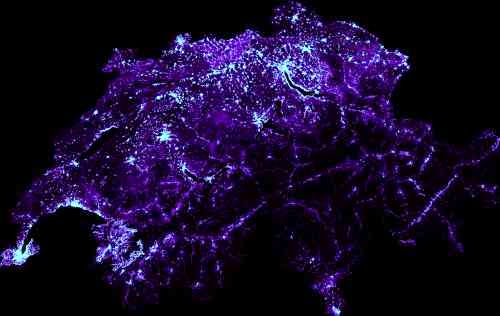## Saturday, October 4, 2014

### An open street map node density map for Switzerland

I'd like to know how open street map nodes are distributed in Switzerland, that is, I want to map their density on an image. This should be a fairly easy task with Python since I have already loaded Switzerland into an sqlite database (See OpenStreetMap: convert an pbf to an sqlite database with Python and download-switzerland-pbf.py [github]).

First, I create a table that contains the count of nodes per pixel. I fill this this table with a `create table... as ...count(*).... group by`. The values for the variables `a` through `f` determine the aspect ration and the size of the resulting picture:

create table count_per_pixel_ch as select count(*) cnt, cast ( ( lon - a ) / c * e as int) x, cast ( ( lat - b ) / d * f as int) y from node group by x, y;

`x` and `y` are unique. This pair represents a pixel on the final image. `cnt` is the number of nodes that fall into the area covered by a `x`/`y` pair.

After filling this table, I can iterate over each pixel and draw a color that is lighter for large pixel counts and darker for small pixel counts. I use the Python Image Library (`import Image`) to draw the image.

import Image # # Code filling the table # for r in cur.execute(""" select x, y, cnt from count_per_pixel_ch where x >= 0 and x < {image_width_px} and y >= 0 and y < {image_height_px} """.format( image_width_px = image_width_px , image_height_px = image_height_px)): x = r y = image_height_px - r - 1 cnt = float(r) blue = int(float(cnt)/float(avg_count_per_pixel) * 255.0) green = 0 if blue > 255: green = blue - 255 blue = 255 pixels[x, y] = (blue/2, green, blue)

Here's the result:The same picture with a better resolution.

Source code on github